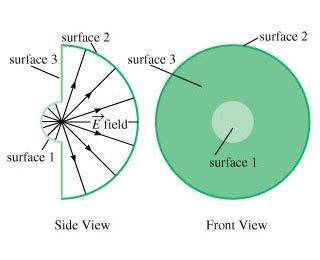# Electric Flux of Two Hemispheres with different radii

Inveritatem

## Homework Statement

Two hemispherical surfaces, 1 and 2, of respective radii r1 and r2, are centered at a point charge and are facing each other so that their edges define an annular ring (surface 3), as shown.## Homework Equations

The field at position r⃗ due to the point charge is:

E⃗ (r⃗ )=C/r^2 r_hat

where C is a constant proportional to the charge, r=∣∣r⃗ ∣∣, and r_hat=r⃗ /r is the unit vector in the radial direction.

## The Attempt at a Solution

The hints imply that because the electric field lines everywhere are parallel to the annular ring (surface 3), the angle between the area and electric field vector is 90, which implies that the flux through surface 3 is 0. I am confused as to why this is the case, as looking at the picture I only see one possibility:

From the side view, the electric field lines seem to only go through surface 1, meaning no electric field passes the surface of surface 3, which leads to 0 electrical flux.

Can anyone help explain why there are electrical field lines that form a 90 degree angle with the area vector?